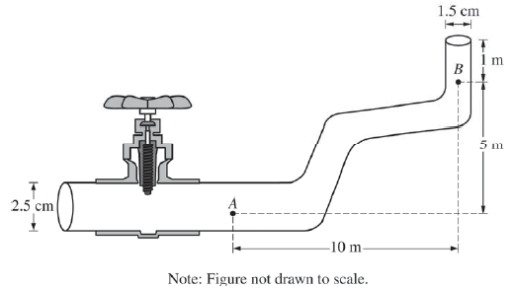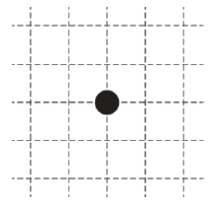# 2017 AP Physics 2 物理Algebra-based真题系列之简答题免费下载

### 真题与答案下载### 2017 AP 物理2简答题部分免费下载

#### 部分真题预览：1）Two students observe water flowing from left to right through the section of pipe shown above, which decreases in diameter and increases in elevation. The pipe ends on the right, where the water exits vertically. At point A the water is known to have a speed of 0.50 m/s and a pressure of 2.0 ×105 Pa to the power 5 pascals . The density of water is
1000 kg/m3.

1. The students disagree about the water pressure and speed at point B. They make the following claims. Student Y claims that the pressure at point B is greater than that at point A because the water is moving faster at point B.
Student Z claims the speed of the water is less at point B than that at point A because by conservation of energy, some of the water’s kinetic energy has been converted to potential energy of the Earth-water system.

1. Indicate any aspects of student Y’s claim that are correct.
2. Indicate any aspects of student Y’s claim that are incorrect. Support your answer using appropriate physics principles.
3. Indicate any aspects of student Z’s claim that are correct.
4. Indicate any aspects of student Z’s claim that are incorrect. Support your answer using appropriate physics principles.
2. Calculate the following at point B.
1. The speed of the water
2. The pressure in the pipe
3. A valve to the left of point A now closes off that end of the pipe. The section of pipe shown is still full of water, but the water is no longer flowing.
1. Calculate the absolute pressure at point A (the pressure that includes the effect of the atmosphere).
2. An air bubble forms at point A. On the figure below, where the dot represents the air bubble, draw a free-body diagram showing and labeling the forces (not components) exerted on the bubble. Draw the relative lengths of all vectors to reflect the relative magnitudes of the forces.### 2017 AP 物理2模考简答题部分免费下载